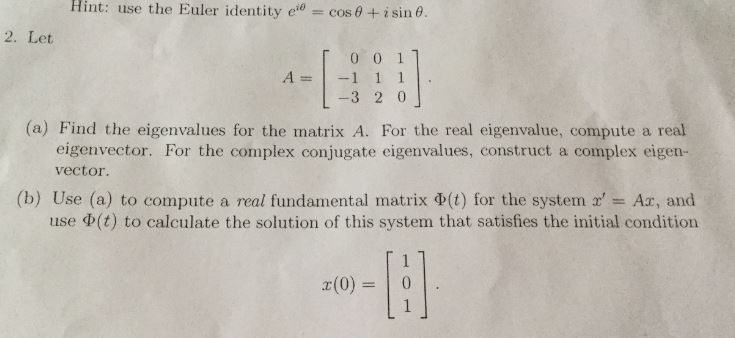Hint: use the Euler identity ecos+isin 62. LetT0 0 1-3 2 0eigenvector. For the complex conjugate eigenvalues, construct a complex eigen-(a) Find the eigenvalues for the matrix A. For the real eigenvalue, compute a realvector.Ax, and(b) Use (a) to compute a real fundamental matrix Φ(t) for the systern r,use Φ(t) to calculate the solution of this system that satisfies the initial condition

Question

pls explain to me step by step. thankshelp_outlineImage TranscriptioncloseHint: use the Euler identity ecos +isin 6 2. Let T0 0 1 -3 2 0 eigenvector. For the complex conjugate eigenvalues, construct a complex eigen- (a) Find the eigenvalues for the matrix A. For the real eigenvalue, compute a real vector. Ax, and (b) Use (a) to compute a real fundamental matrix Φ(t) for the systern r, use Φ(t) to calculate the solution of this system that satisfies the initial condition fullscreen
Step 1
Step 2
Step 3

Want to see the full answer?

See Solution

Want to see this answer and more?

Our solutions are written by experts, many with advanced degrees, and available 24/7

See Solution
Tagged in

Math